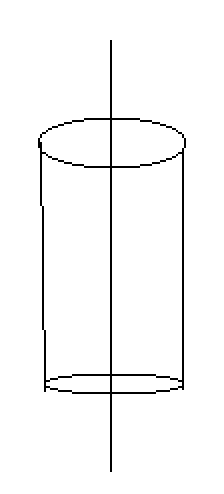# Trying to derive Gauss' law using a cylindrical surface

• I
• annamal

#### annamal

When I try to derive Gauss's law with a straight line of charge with density ##\lambda## through a cylindrical surface of length L and radius R,
$$\vec E = \frac{\lambda*L}{4\pi\epsilon*r^2}$$
$$A = 2\pi*r*L$$
$$\vec E*A = \frac{\lambda *L^2}{2\epsilon*r} \neq \frac{q_{enc}}{\epsilon}$$
What am I doing wrong?$$\vec E = \frac{\lambda*L}{4\pi\epsilon*r^2}$$
$$\int_{\partial V} \mathrm{d}^3 \vec{f} \cdot \vec{E}=\frac{1}{\epsilon_0} Q_V,$$